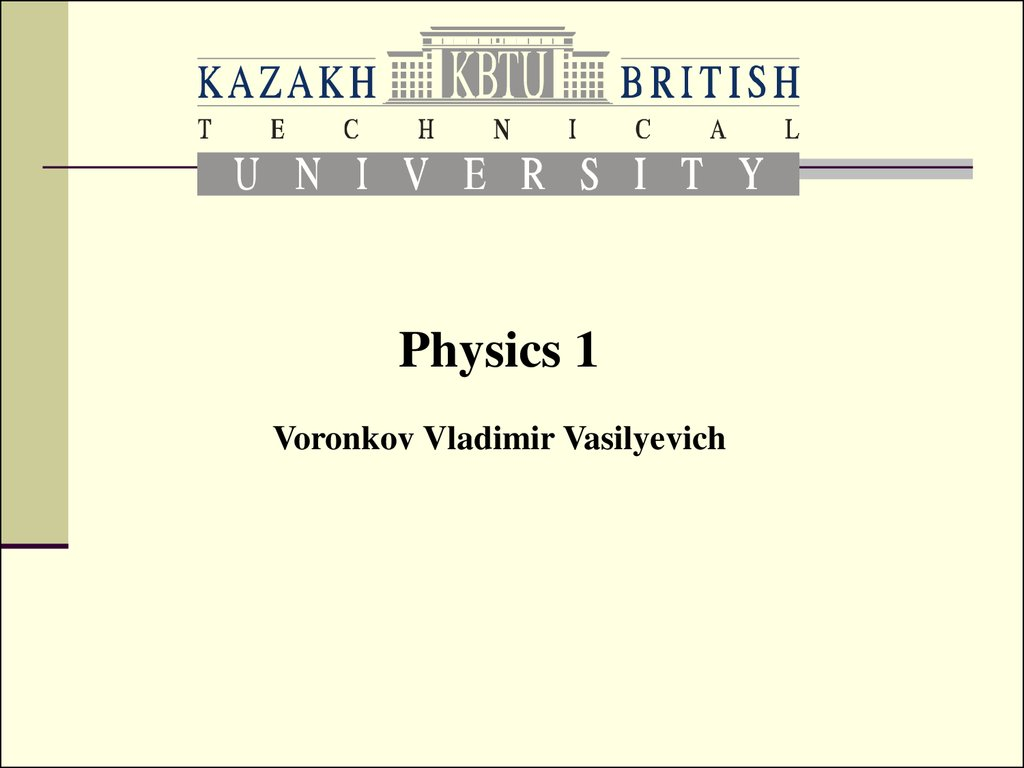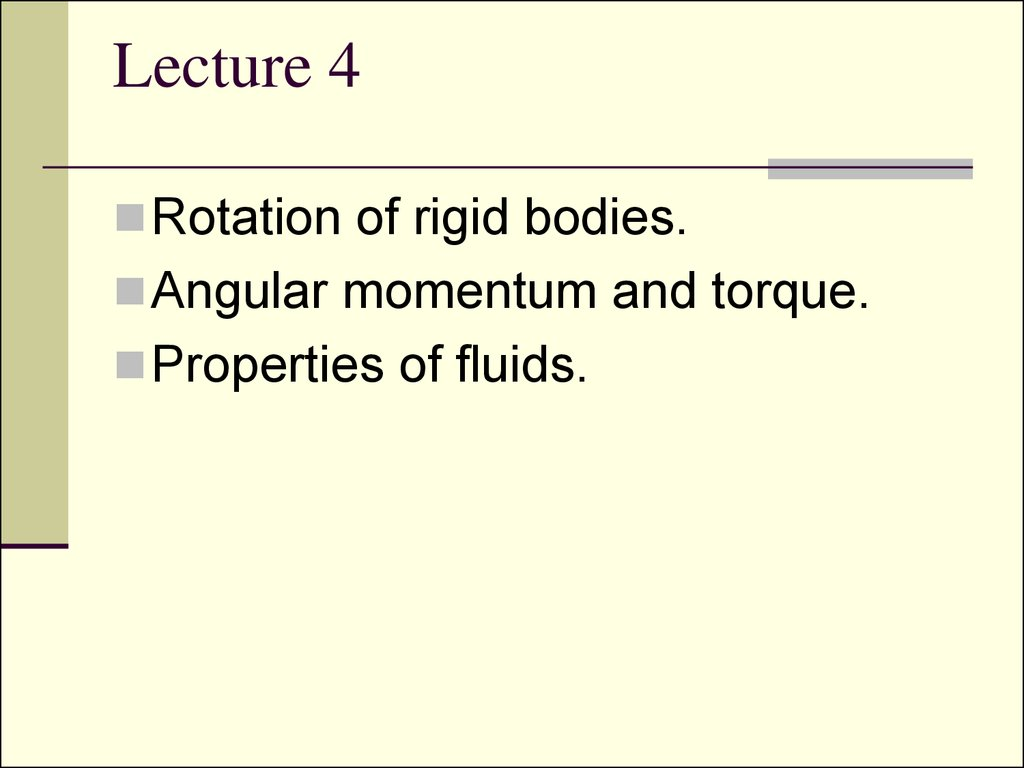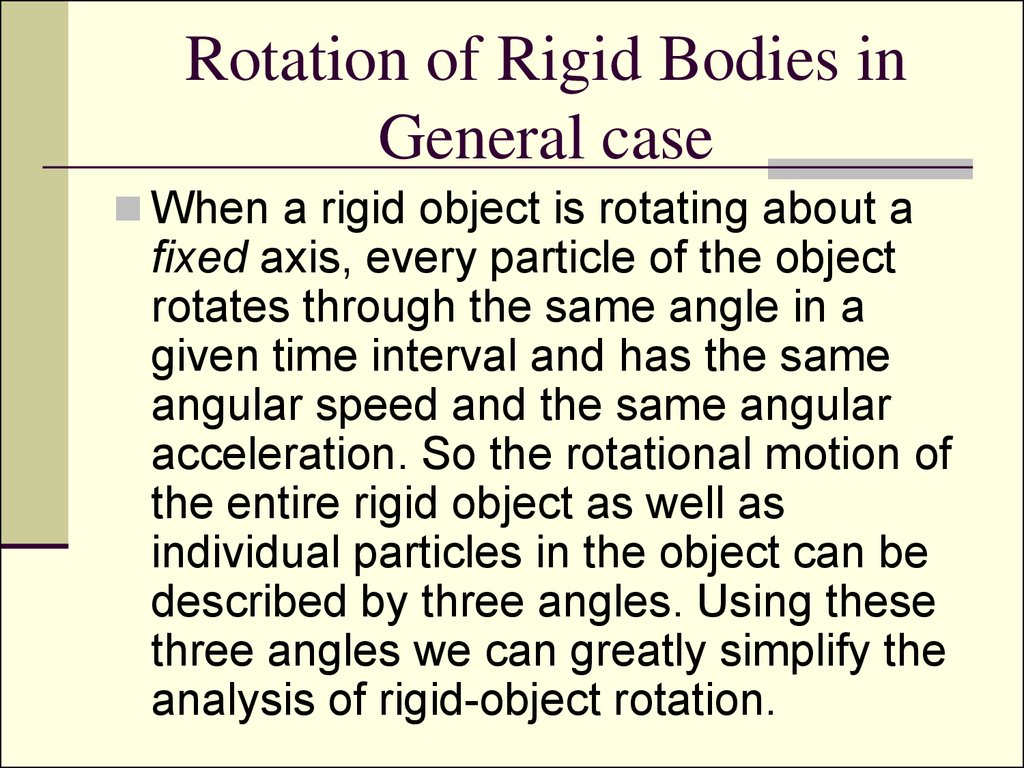# Rotation of rigid bodies. Angular momentum and torque. Properties of fluids

Physics 1

## 2. Lecture 4

Rotation of rigid bodies.
Angular momentum and torque.
Properties of fluids.

## 3. Rotation of Rigid Bodies in General case

When a rigid object is rotating about a
fixed axis, every particle of the object
rotates through the same angle in a
given time interval and has the same
angular speed and the same angular
acceleration. So the rotational motion of
the entire rigid object as well as
individual particles in the object can be
described by three angles. Using these
three angles we can greatly simplify the
analysis of rigid-object rotation.

ratio of the arc length s and the

## 5. Angular kinematics

Angular displacement:
Instantaneous angular
speed:
Instantaneous angular
acceleration:

## 6. Angular and linear quantities

Every particle of the object moves in a circle
whose center is the axis of rotation.
Linear velocity:
Tangential acceleration:
Centripetal acceleration:

## 7. Total linear acceleration

Tangential acceleration is perpendicular to
the centripetal one, so the magnitude of total
linear acceleration is

## 8. Angular velocity

Angular velocity is a vector.
The right hand rule
is applied: If the
hand curl along
with the rotation
give the direction o
the angular
velocity.

## 9. Rotational Kinetic Energy

Moment of rotational inertia
Rotational kinetic energy

## 16. Parallel-axis theorem

Suppose the moment of inertia about an axis
through the center of mass of an object is ICM.
Then the moment of inertia about any axis
parallel to and a distance D away from this
axis is

## 18. Torque

When a force is exerted on a rigid object pivoted
that axis. The tendency of a force to rotate an
object about some axis is measured by a vector
quantity called torque t (Greek tau).

## 19.

The force F has a greater rotating tendency
about axis O as F increases and as the
moment arm d increases. The component F
sinf tends to rotate the wrench about axis O.

## 20.

The force F1 tends to rotate the
and F2 tends to rotate it clockwise.
We use the convention that the sign of the
torque resulting from a force is positive if the
turning tendency of the force is
counterclockwise and is negative if the
turning tendency is clockwise. Then

## 21. Torque is not Force Torque is not Work

Torque should not be confused with force. Forces can
cause a change in linear motion, as described by
Newton’s second law. Forces can also cause a change
in rotational motion, but the effectiveness of the forces in
causing this change depends on both the forces and the
moment arms of the forces, in the combination that we
call torque. Torque has units of force times length:
newton · meters in SI units, and should be reported in
these units.
Do not confuse torque and work, which have the same
units but are very different concepts.

## 22. Rotational Dynamics

and
Then:
which equals zero, as
are parallel.
So we get

## 23. Rotational analogue of Newton’s second law

Quantity L is an instantaneous
angular momentum.
The torque acting on a particle is
equal to the time rate of change of
the particle’s angular momentum.

## 24. Net External Torque

The net external torque acting on a
through an origin in an inertial frame
equals the time rate of change of the
total angular momentum of the system

## 25. Angular Momentum of a Rotating Rigid Object

Angular momentum for each particle of an
object:
Angular momentum for the whole object:
Thus:

## 27. The Law of Angular Momentum Conservation

The total
angular momentum of a
system is constant if the resultant
external torque acting on the system is
zero, that is, if the system is isolated.

## 28.

Change in internal structure of a rotating body
can result in change of its angular velocity.

## 29.

When a rotating skater pulls his hands towards
his body he spins faster.

Full mechanical
energy, linear
momentum and
angular
momentum of an
isolated system
remain constant.

## 31. Work-Kinetic Theory for Rotations

Similarly to linear motion:

## 32.

The net work done by external
forces in rotating a symmetric rigid
object about a fixed axis equals the
change in the object’s rotational
energy.

## 34. Independent Study for IHW2

Vector multiplication (through their components
i,j,k).Right-hand rule of Vector multiplication.
2. Elasticity
1.
1.
2.
Demonstrate by example and discussion your
understanding of elasticity, elastic limit, stress,
strain, and ultimate strength.
Write and apply formulas for calculating Young’s
modulus, shear modulus, and bulk modulus. Units
of stress.

## 35.

3.
Fluids
1.
2.
3.
4.
5.
6.
Define absolute pressure, gauge pressure, and
atmospheric pressure, and demonstrate by
relationships between these terms.
Pascal’s law.
Archimedes’s law.
Rate of flow of a fluid.
Bernoulli’s equation.
Torricelli’s theorem.

## 36. Literature to Independent Study

Lecture on Physics Summary by Umarov.
(Intranet)
2. Fishbane Physics for Scientists… (Intranet)
3. Serway Physics for Scientists… (Intranet)
1.

## 37. Problems

1. A solid sphere and a hollow sphere have the
same mass and radius. Which momentum of
rotational inertia is higher if it is? Prove your
2. What are the units for, are these quantities
vectors or scalars:
1.
2.
3.
4.
5.
6.
Angular momentum
Angular kinetic energy
Angular displacement
Tangential acceleration
Angular acceleration
Torque